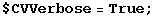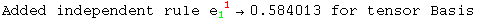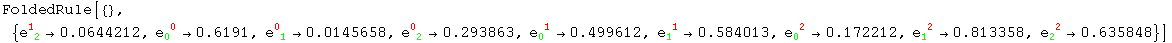7.6. BasisValues

BasisValues        Values for the components of basis changes

Components of the basis changes

The components of the basis change matrices require separate treatment and are represented by the BasisValues head. Here we do not allow derivatives, because the derivatives of these objects are automatically replaced by Christoffel tensors.

In:=In:=In:=Out=In:=Out=In:=Out=The inverse basis change is not automatically computed, because it may take a very long time

In:=Out=Created by Mathematica  (May 16, 2008)